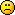Discussion about math, puzzles, games and fun.   Useful symbols: ÷ × ½ √ ∞ ≠ ≤ ≥ ≈ ⇒ ± ∈ Δ θ ∴ ∑ ∫  π  -¹ ² ³ °

You are not logged in.

## #1 2020-07-03 03:13:59

pi_cubed
Member
From: A rhombicosidodecahedron
Registered: 2020-06-22
Posts: 61

### Simple VBScript Programs

Here are some simple VBScript programs.
Note: I originally had a detailed explanation for each one but this page suddenly reloaded and I don't want to retype everything. I'll update it later. Also, the size of the message sending input box is different from the viewing message box, so sorry if there are single quotes for new lines of comments where they aren't supposed to be and if one line spills into the other.

``````'These are comments in VBScript
'Strings must be in double quotes "" not single quotes ' '
'1. Msgbox
x=msgbox("Hi there", 1, "Title")
'Note: The 1 is a number that denotes the type of msgbox. It can also be some non-string text, such as vbYesNo. 'Please google it for more info
'2. Inputbox
x=inputbox("question/text", "title")
'3. Quiz with if else Elseif
a=inputbox("type yes or no for yes and maybe for no, case-sensitive", "Quiz")
dim score : score = 0
'Create a variable and define a value
if a="yes" or a="no" then
j=msgbox "yes"
score = score+1
'Set score = score+1 also works
elseif a="maybe" then
k=msgbox "no"
else
l=msgbox "I don't understand."
'Extra: Looping
dim var : var = 0
do until var = 11
x=msgbox(var, "loop")
loop
'Warning: the following is an infinite loop. In some cases it can be stopped from the task manager, in some cases 'you will have to shutdown and restart your computer
do
message=msgbox("infinite loop", "title: infinite loop"
loop``````
pi_cubed wrote:

Tell me if anything is wrong.

Last edited by pi_cubed (2020-07-03 03:18:20)

e to the i pi plus 1 is zero -Leonhard Euler | a squared plus b squared equals c squared -Pythagoras | Energy equals mass times the speed of light squared -Albert Einstein | i² = -1 | x^2+1=0 x^2=-1 x=i

Offline